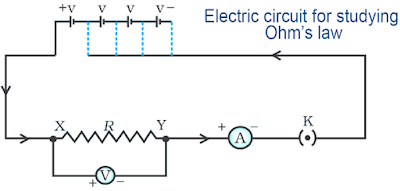# Electricity | Activities in Text Book with Solution

Activity 12.1

Set up a circuit as shown in Fig. 12.2, consisting of a nichrome wire XY of length, say 0.5 m,
an ammeter, a voltmeter and four cells of 1.5 V each. (Nichrome is an alloy of nickel, chromium,
manganese, and iron metals.)Fig. 12.2

First use only one cell as the source in the circuit. Note the reading in the ammeter I, for the current and reading of the voltmeter V for the potential difference across the nichrome wire XY in the circuit. Tabulate them in the Table given.

Next connect two cells in the circuit and note the respective readings of the ammeter and voltmeter for the values of current through the nichrome wire and potential difference across the nichrome wire.

Repeat the above steps using three cells and then four cells in the circuit separately.

Calculate the ratio of V to I for each pair of potential difference V and current I.

 Number of cells used Current through the nichrome wire, I (ampere) Potential difference across nichrome wire, V (volt) V/I (volt/ ampere) 1 2 3 4

Plot a graph between V and I, and observe the nature of the graph.